Courses
Courses for Kids
Free study material
Free LIVE classes
More

# Functional Equations - Detailed Explanation with Methods for JEELIVE
Join Vedantu’s FREE Mastercalss

## What is Meant by Functional Equations?

The term “functional equations” comprises two individual terms: functions and equations. Functions are essentially any polynomial expression that establishes the relationship between linear, cubic or contains polynomial expressions. In fact, there are many types of functions in Mathematics, which also include logarithmic functions, sin and cosine functions, exponential functions, cyclic functions, and many more.  In the case of functional equations, the unknown variables are basically unknown functions, and therefore the methods of segregating the unknown values are very different from those of the conventional methods of solving an equation and finding the values of the unknown variables.

## The Different Types of Functions in Mathematics

As mentioned before, there are a variety of different types of functions that we come across in the field of Mathematics. Every function has its own standard form and can be altered slightly by keeping the fundamental form as the original. The following list shows the different types of functions and functional equations in their general form. Here, we consider the notations f(x) and g(x) as per convention

 Sr. No. Mathematical term Formula Graph 1. linear function f(x) = mx +c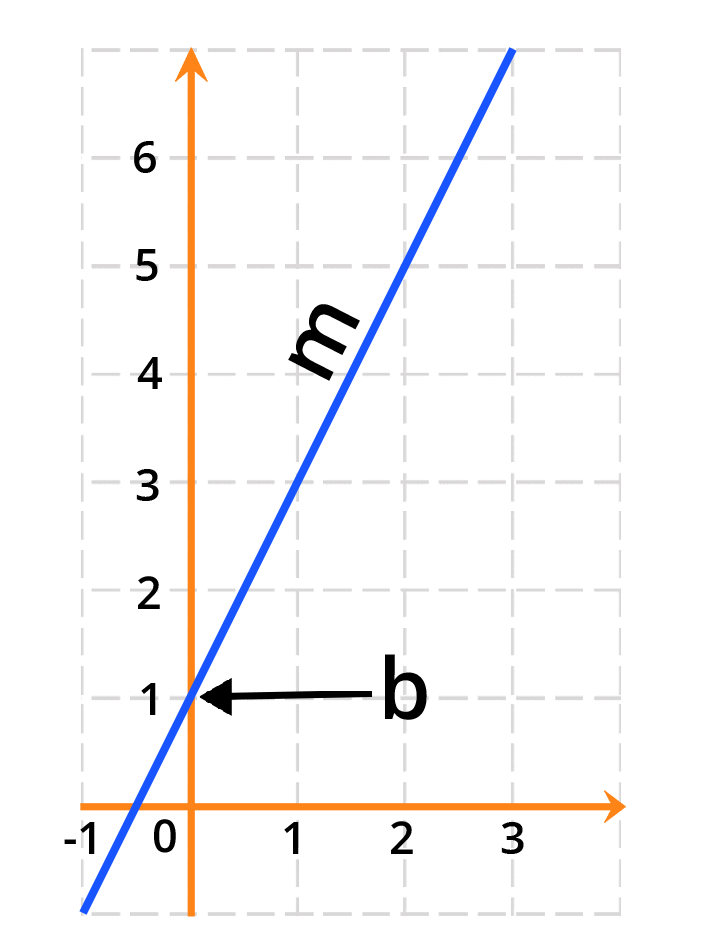2. Quadratic function f(x) = x2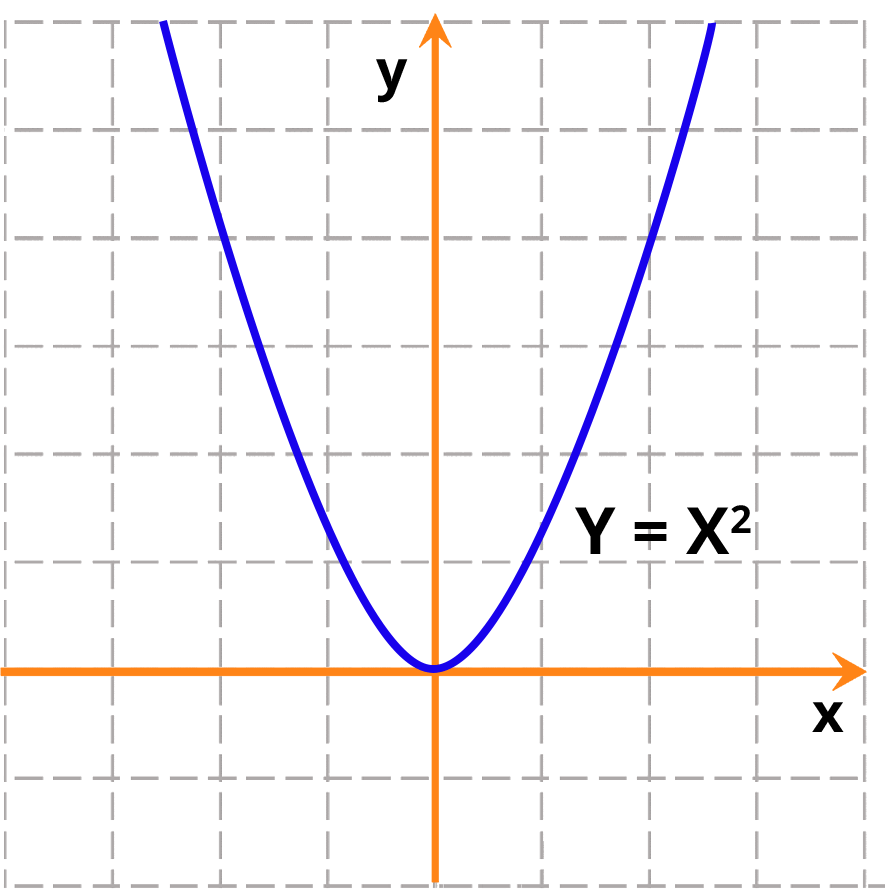3. Square root function $f(x)=\sqrt{x}$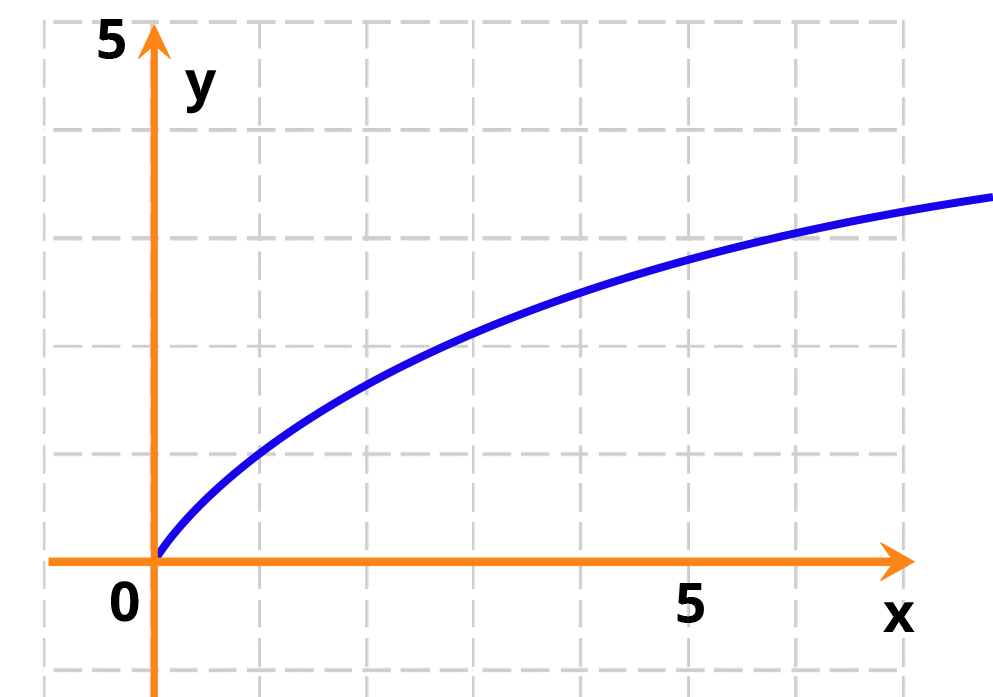4. Absolute value function f(x) = |x|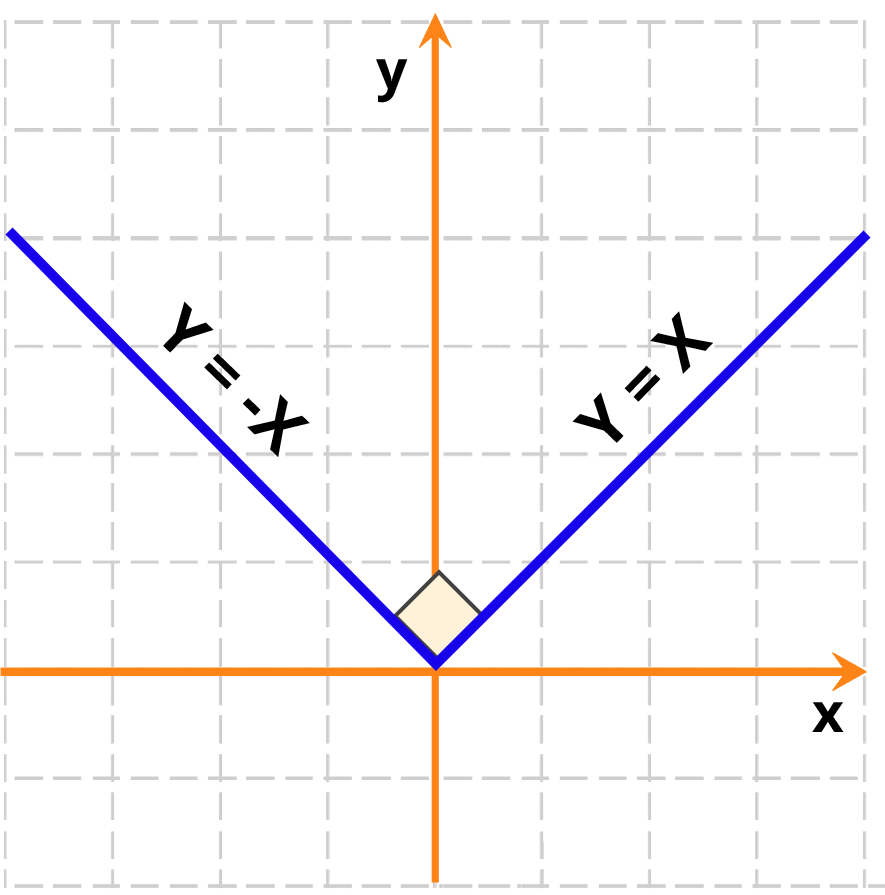5. Reciprocal function $f(x)=\dfrac{1}{x}$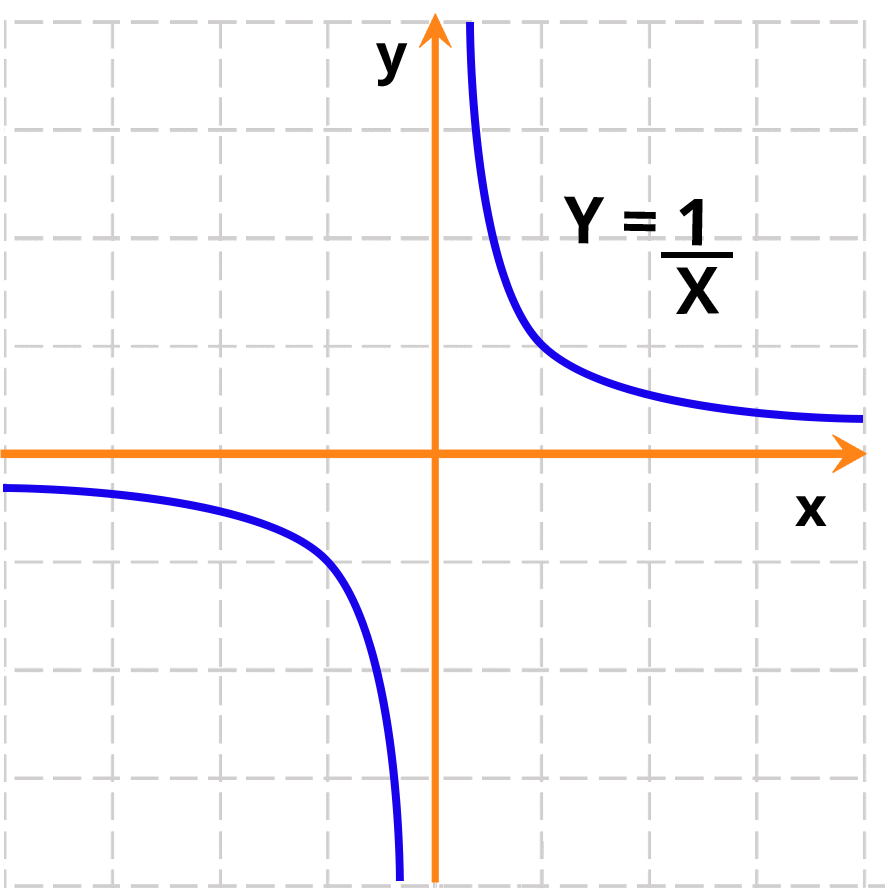6. Logarithmic function f(x) = ln x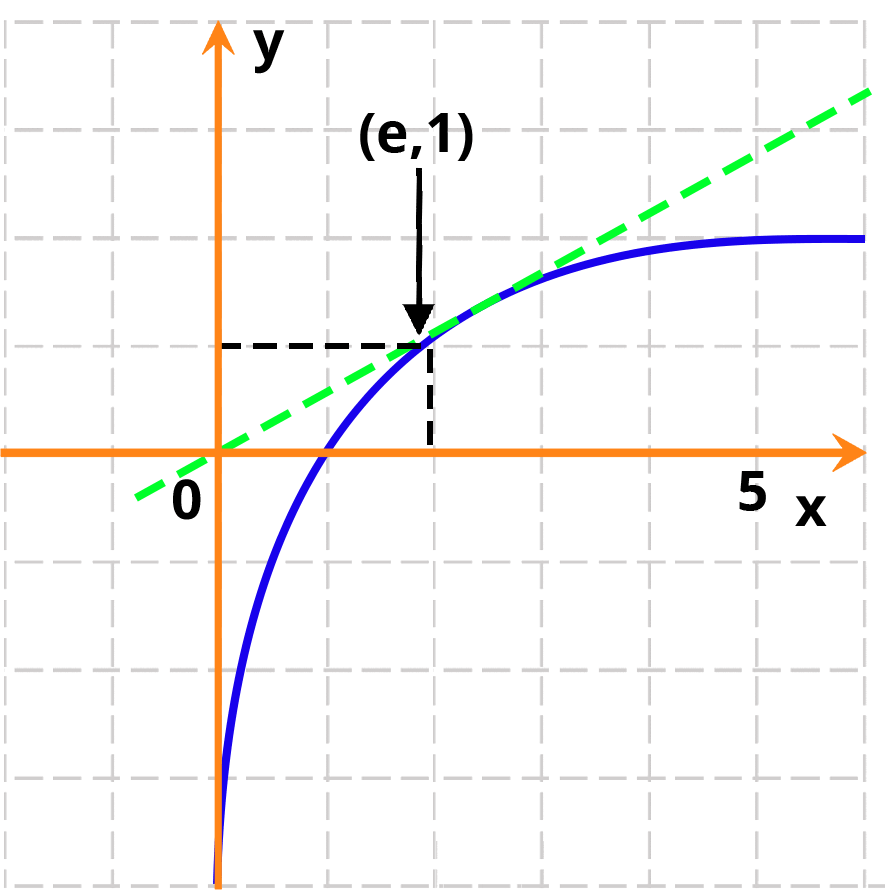7 Exponential function f(x)=ex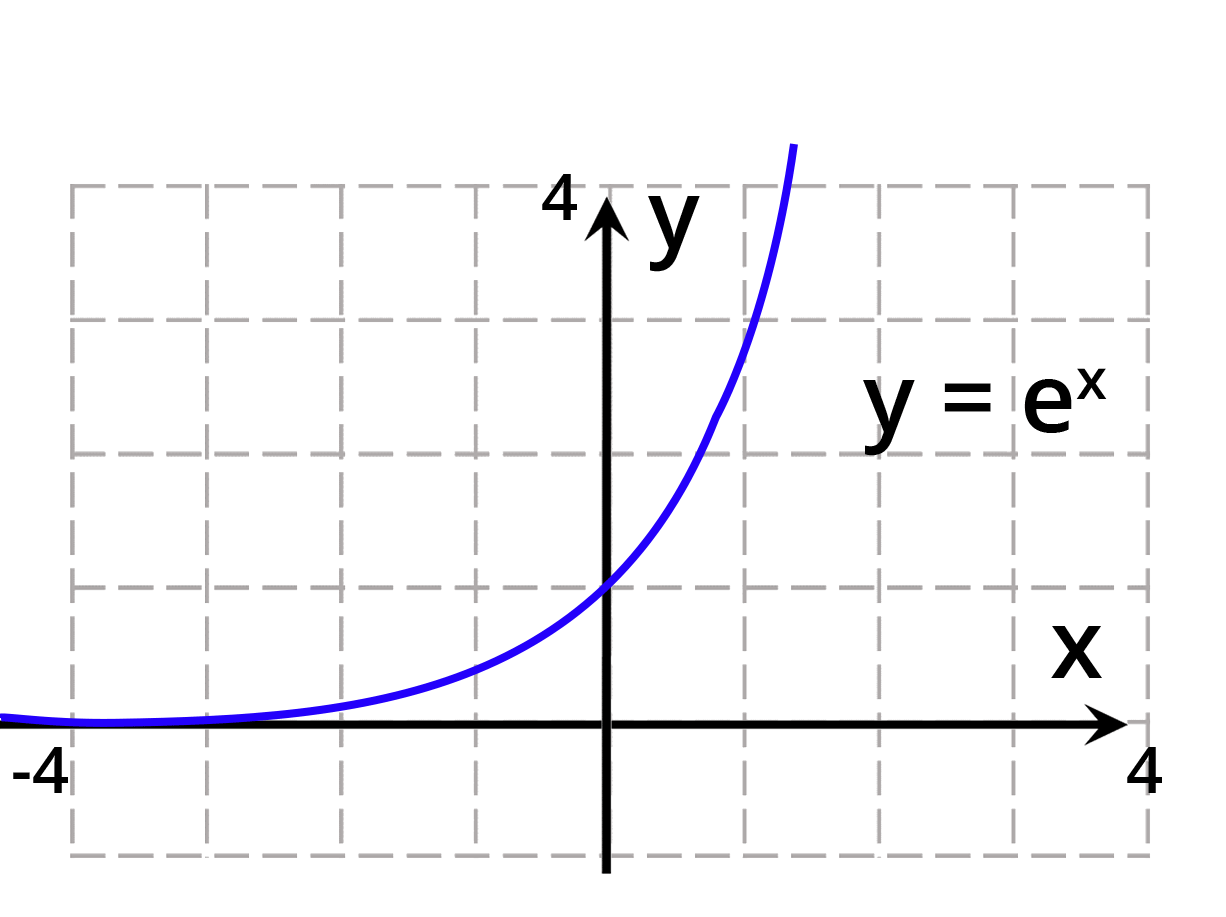8. Sin function f (x) = sin (x)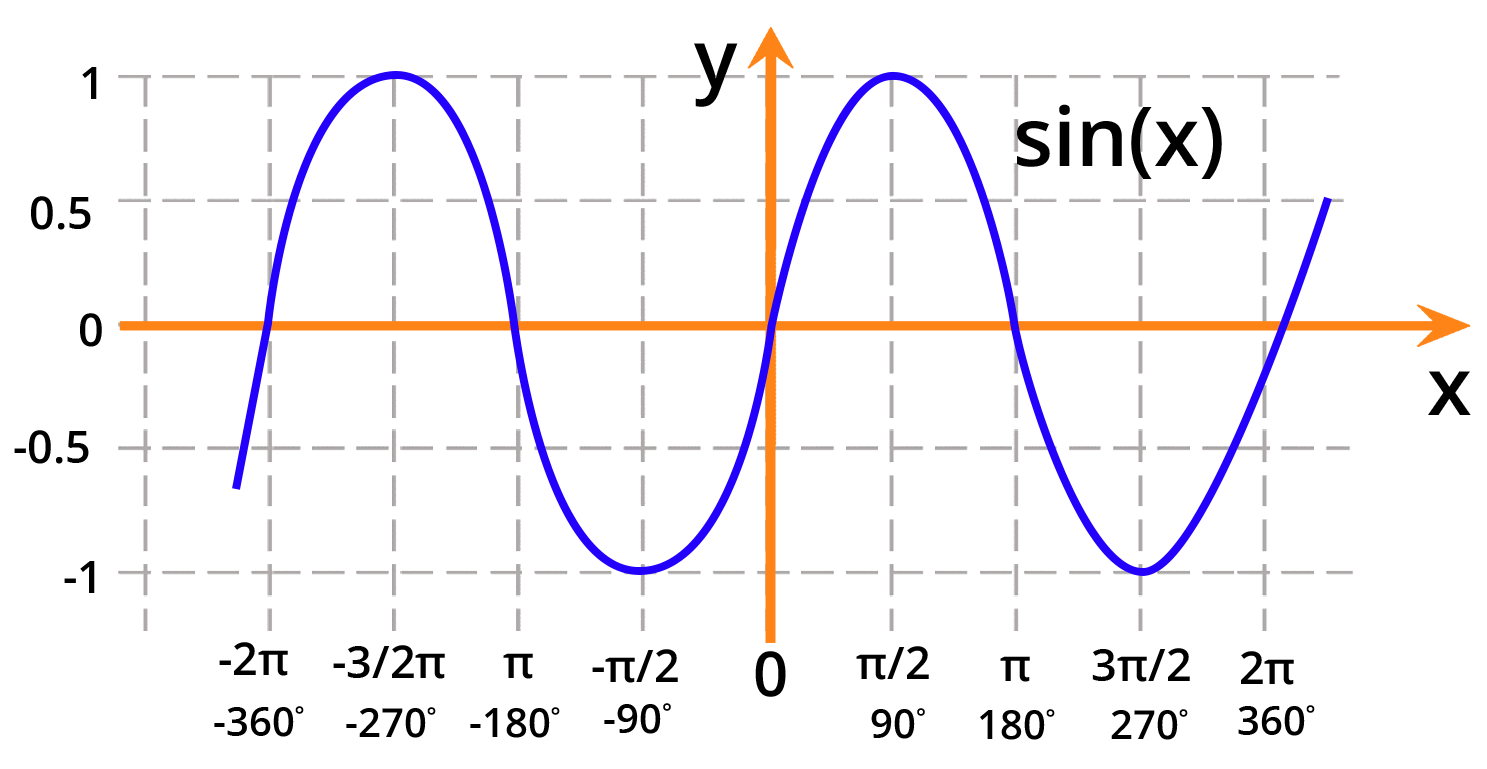9. Cosine function f (x) = cos (x)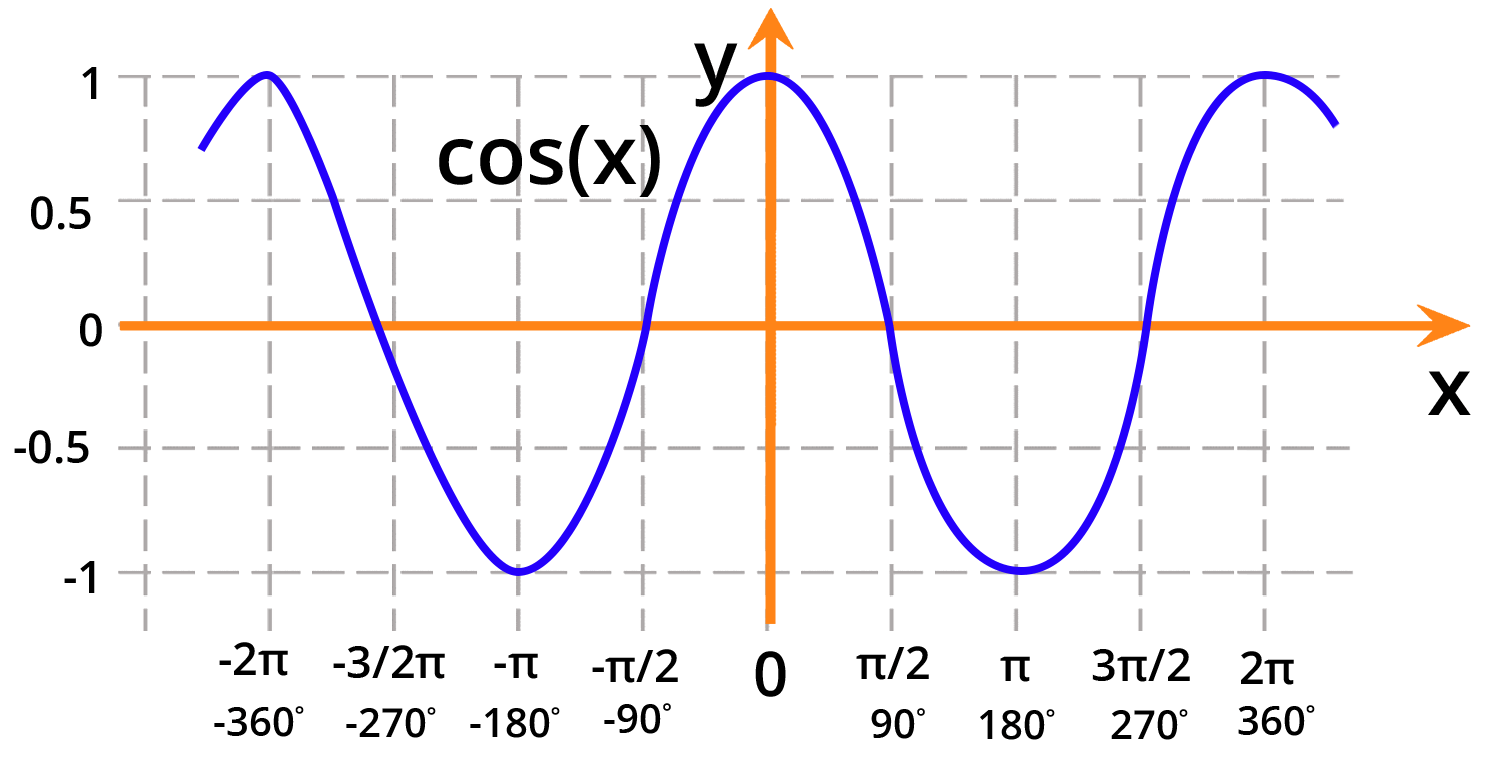10. Tan function f (x) = tan (x)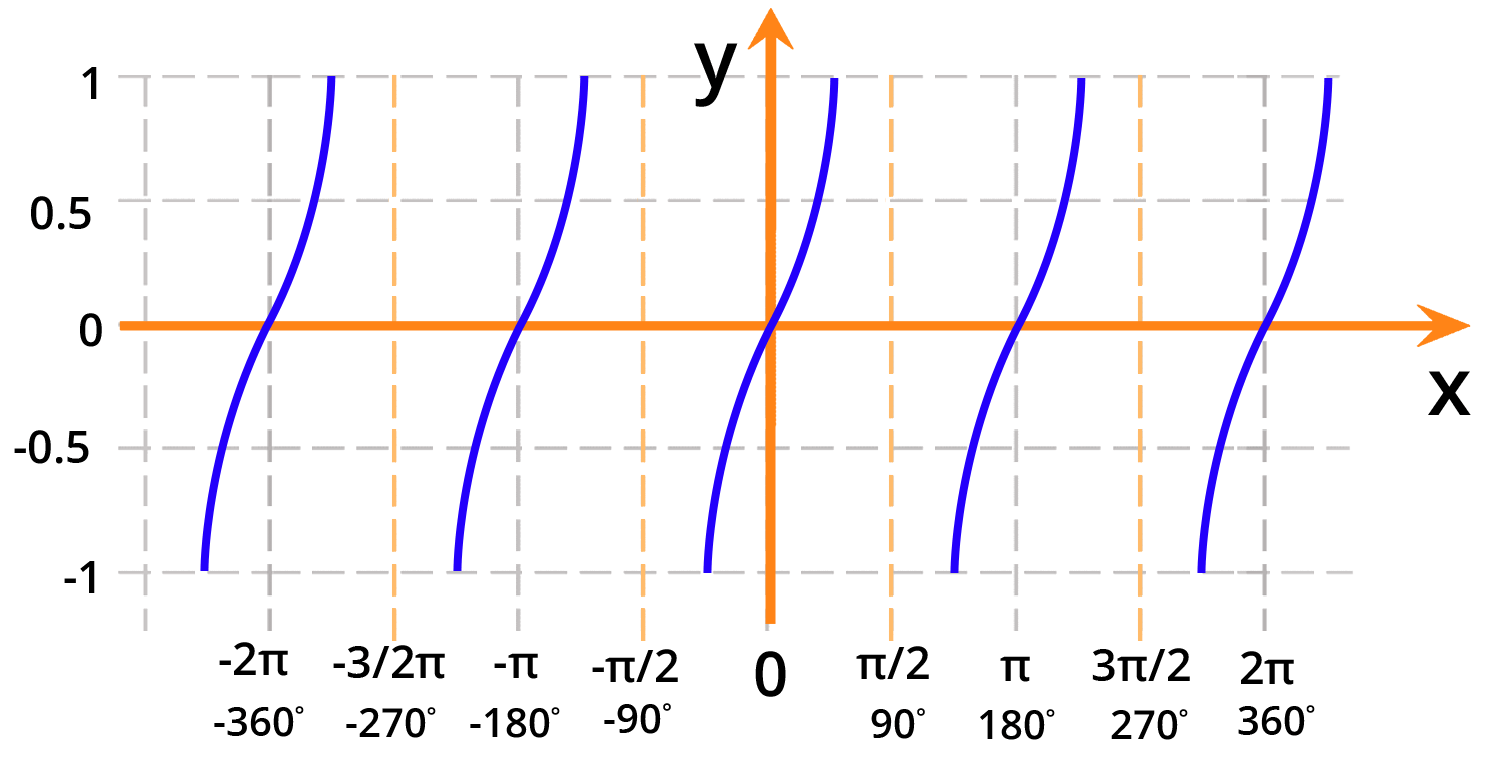11. Step function or the greatest integer function ( floor function) f(x) = ⌈ x ⌉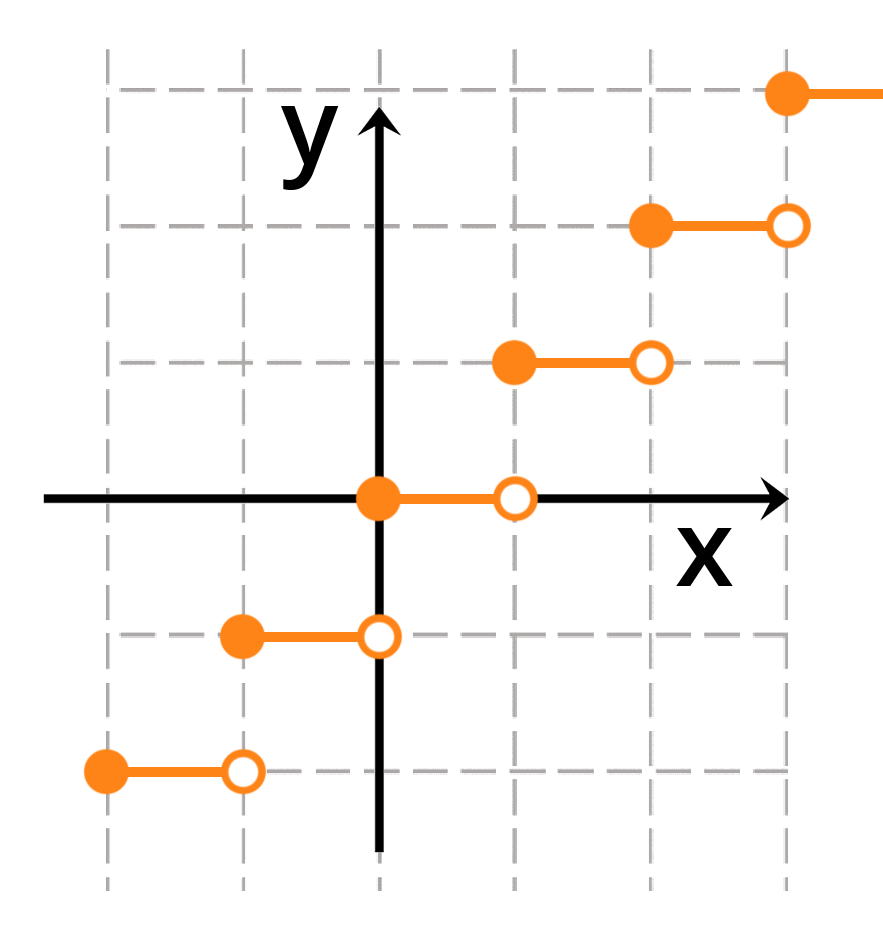12. Step function or the greatest integer function ( ceiling function) f (x) = ⌊ x ⌋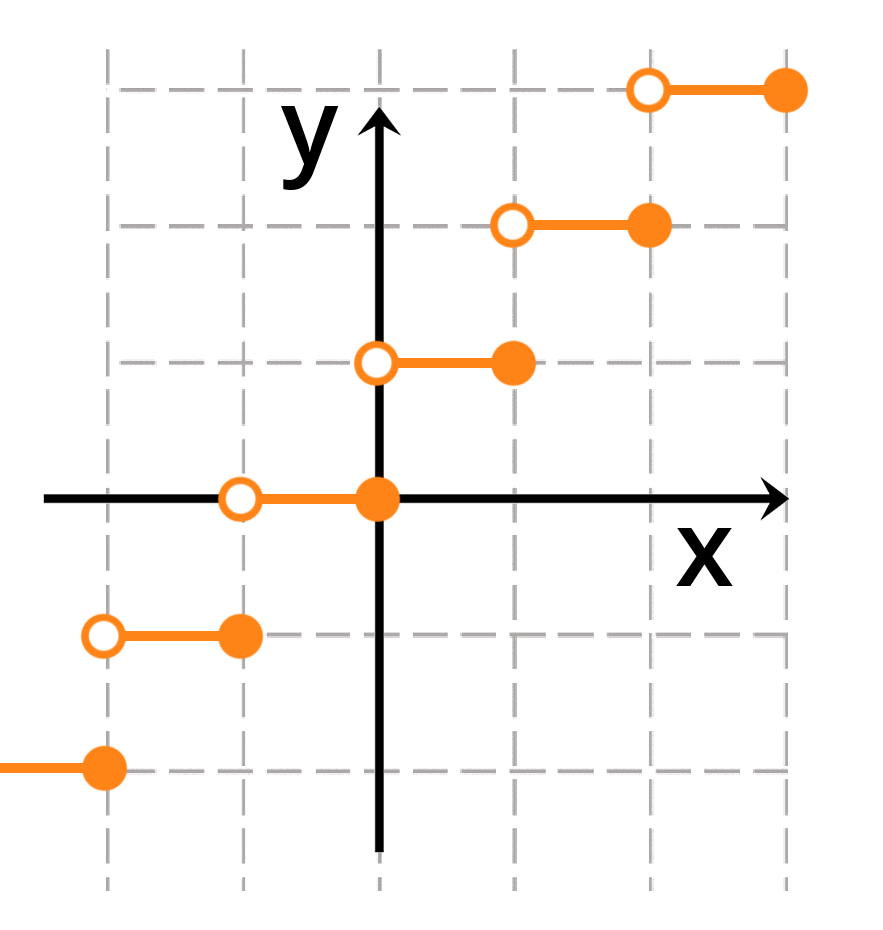## Basic Method for Finding the Functional Relationship

### There are some other methods which can effectively solve a functional equation or can be implemented to find the functional relationships. They are as follows:

1. Surjective Function/Onto Function: Between sets X and Y, a surjective function is constructed such that every element of set Y is related with at least one element of set X. A surjective function's domain and range are both the same.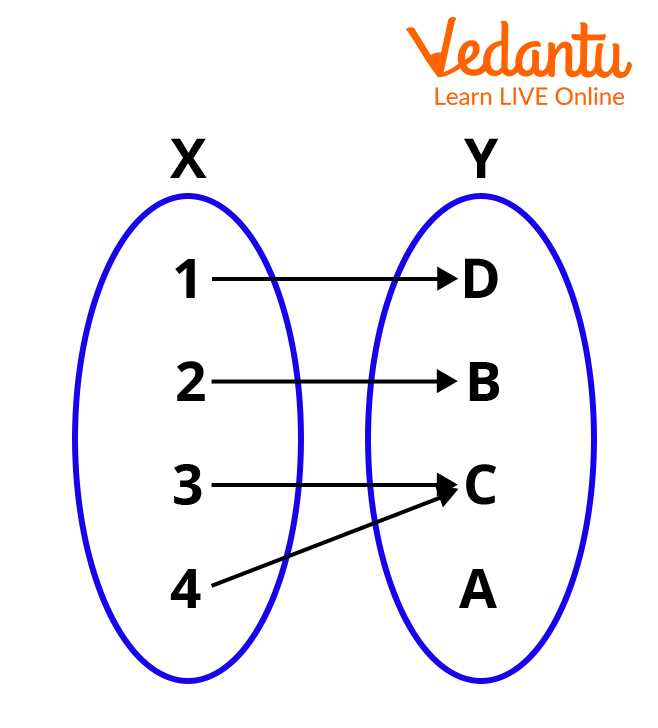Example:

Let, $X=\{1,2,3,4\},~Y=\{A, B, C, D\}$

$f: A \rightarrow B$

2. Injective Function/One to One Function:  In Injective function, the elements  of the two sets are mapped so that every element of the range is in co-domain and has a distinct domain element. If f is both one-one or injective and onto or surjective, it is said to be a bijective function or bijection.

Example:

Let, $A=\{X, Y, Z, W\},~B=\{1,2,3,5\}$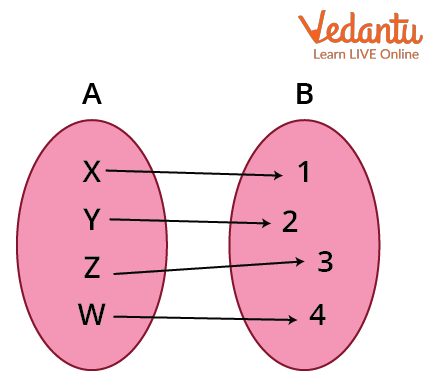## Examples of  Finding Functional Relationships

Let us take the following examples:

Example 1: If $f(x+3)=x^{2}+8 x+16$, then find the value of f(x).

Solution: Here, as we can see, the function f(x+3) has been given. From the given function, we must establish a relationship between f(x+3) and f(x) to find out the value of f(x). We apply the method of substitution to do the same.

Let us substitute x+3 with x-3 such that x = (x-3) + 3

Substituting the value of y in the given equation and simplifying, we get that:

\begin{align} &f(x+3)=x^{2}+8 x+16 \\ &\Rightarrow f((x-3)+3)=f(x) \\ &\Rightarrow f(x)=(x-3)^{2}+8(x-3)+16 \\ &=x^{2}+9-6 x+8 x-24+16 \\ &=x^{2}+2 x+1 \text { or }(x+1)^{2} \end{align}

Therefore, the required expression for f(x) is $f(x)=x^{2}+2 x+1=(x+1)^{2}$.

Thus, we not only found the value of f(x) from f(x+3) but also derived a relationship between the two functional equations.

Example 2: Find all the functions $f: R-\{0\} \rightarrow R$ satisfying the equation $f(x)+3 f\left(\dfrac{1}{x}\right)=x^{2}$

Solution:

Let us substitute $\left(\dfrac{1}{x}\right)$ in the function for a single variable. However, as mentioned in the theory of equations, a function or a polynomial expression can have one or more variables. Using the method of substitution to solve functional equations having more than one unknown variable can get a little confusing. The one key point that you must remember to check is symmetry. In many cases, just interchanging the variables can establish a symmetrical relationship between the variables of the equation that was not there priorly.

## Conclusion

Functional equations are a system of equations that do not deal with the concept of traditional one or more variables. Rather, these equations consist of unknown functions in the place of variables. The basic method gives us different techniques and approaches that can be used to solve an equation.

Functional equations, on the other hand, cannot be solved by implementing these go-to methods. One of the basic methods for finding the functional relationship for a system of functional equations is substitutions. Cos function, the sine function is also exhibited in the physical world apart from Mathematics.

Last updated date: 22nd Sep 2023
Total views: 133.2k
Views today: 2.33k

## FAQs on Functional Equations - Detailed Explanation with Methods for JEE

1. What are the different types of functions found in Maths?

In Mathematics, there are many different types of functions. Some of the most frequent mathematical functions include linear, quadratic, cubic, onto, into, one-to-one, many-to-one, trigonometric, logarithmic, and exponential functions. Another distinction that tells us how the two sets holding the function's input and output are related is subjective, bijective which is both, injective and surjective.

2. What significance does the current issue have in terms of board exams? How much weight does this topic have in the JEE exam?

In the board exam, the current topic of functional equations has intermediate levels. Since the board test is subjective, the theoretical and numerical sections of this text are critical from an exam standpoint. Some numerical information is also available from the score advantage section.

The weightage of the functional equations is moderate because it only comprises a small amount of Mathematics. The parabola is a simple idea that is used in this topic. Every year 2 to 3 questions from functional equations are asked in the